# Electronics and Communication Engineering - Networks Analysis and Synthesis

16.

A series RC circuit has R = 5 Ω and C = 10 μF. The current in the circuit is 5 sin 20000t. The applied voltage is

 A. 252 sin (20000t + 45°) B. 252 sin (20000t - 45°) C. 252 sin 20000t D. 252 sin (20000t - 90°)

Explanation: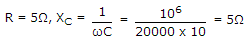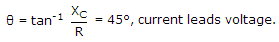Z = R2 + XC2 = 50

V = 550 sin(20000 t - 45°)

V = 252 sin(20000 t - 45°)

17.

A capacitor stores 0.15C at 5 V. Its capacitance is

 A. 0.75 F B. 0.75 μF C. 0.03 F D. 0.03 μF

Explanation:

Q = CV or 0.15 = C(5) or C = 0.03 F.

18.

A 0.5 μF capacitor is connected across a 10 V battery. After a long time, the circuit current and voltage across capacitor will be

 A. 0.5 A and 0 V B. 0 A and 10 V C. 20 A and 5 V D. 0.05 A and 5 V

Explanation:

When the capacitor is fully charged, i = 0 and voltage across capacitor is equal to battery voltage.

19.

For node 1 in figure, KCL equation is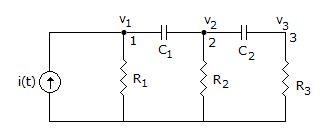A.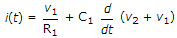B.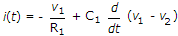C.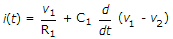D. none of the above

Explanation:

i(t) is the incoming current. The currents leaving node 1 are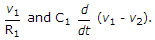20.

In the circuit of figure, the switch is closed at t = 0. At t = 0+ the current through C is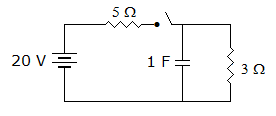A. 4 A B. 2.5 A C. 3.1 A D. 0